# Transitive group

(diff) ← Older revision | Latest revision (diff) | Newer revision → (diff)

A permutation group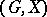such that each elementcan be taken to any element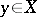by a suitable element, that is,. In other words,is the unique orbit of the group. If the number of orbits is greater than 1, thenis said to be intransitive. The orbits of an intransitive group are sometimes called its domains of transitivity. For an intransitive groupwith orbits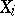,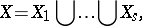and the restriction of the group action tois transitive.

Letbe a subgroup of a groupand let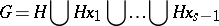be the decomposition ofinto right cosets with respect to. Further, let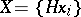. Then the action ofis defined by. This action is transitive and, conversely, every transitive action is of the above type for a suitable subgroupof.

An actionis said to be-transitive,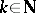, if for any two ordered sets ofdistinct elements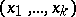and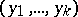,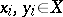, there exists an element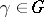such that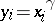for all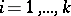. In other words,possesses just one anti-reflexive-orbit. For, a-transitive group is called multiply transitive. An example of a doubly-transitive group is the group of affine transformations,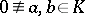, of some field. Examples of triply-transitive groups are the groups of fractional-linear transformations of the projective line over a field, that is, transformations of the form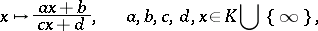whereA-transitive groupis said to be strictly-transitive if only the identity permutation can leavedistinct elements offixed. The group of affine transformations and the group of fractional-linear transformations are examples of strictly doubly- and strictly triply-transitive groups.

The finite symmetric group(acting on) is-transitive. The finite alternating groupis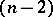-transitive. These two series of multiply-transitive groups are the obvious ones. Two-transitive groups, namelyand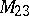, are known, as well as two-transitive groups, namelyand(see  and also Mathieu group). There is the conjecture that apart from these four groups there are no non-trivial-transitive groups for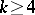. This conjecture has been proved, using the classification of finite simple non-Abelian groups . Furthermore, using the classification of the finite simple groups, the classification of multiply-transitive groups can be considered complete.-Transitive groups have also been defined for fractionalof the form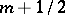,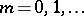. Namely, a permutation groupis said to be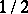-transitive if either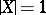, or if all orbits ofhave the same length greater than 1. For, a groupis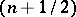-transitive if the stabilizeris-transitive on(see ).

How to Cite This Entry:
Transitive group. Encyclopedia of Mathematics. URL: http://encyclopediaofmath.org/index.php?title=Transitive_group&oldid=17556
This article was adapted from an original article by L.A. Kaluzhnin (originator), which appeared in Encyclopedia of Mathematics - ISBN 1402006098. See original article